# The Vector Class Vector Class Write a Vector

• Slides: 38The Vector ClassVector Class • Write a Vector class. This will be a container class that will hold objects. It should allow the following operations: – add – find – get Add an object to the end of the Vector Determine if an object is in the Vector Get a certain element (by number) from the Vector – insert Insert a new element into a certain position – delete Delete a certain element from the Vector – size Returns size of Vector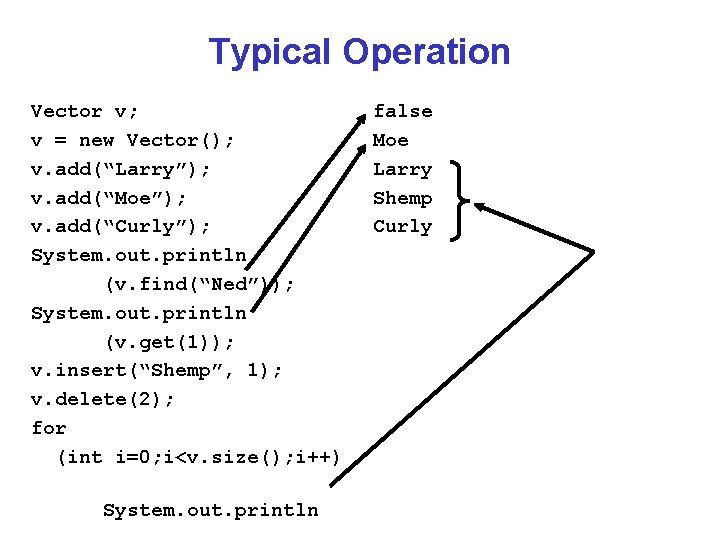Typical Operation Vector v; v = new Vector(); v. add(“Larry”); v. add(“Moe”); v. add(“Curly”); System. out. println (v. find(“Ned”)); System. out. println (v. get(1)); v. insert(“Shemp”, 1); v. delete(2); for (int i=0; i<v. size(); i++) System. out. println false Moe Larry Shemp Curly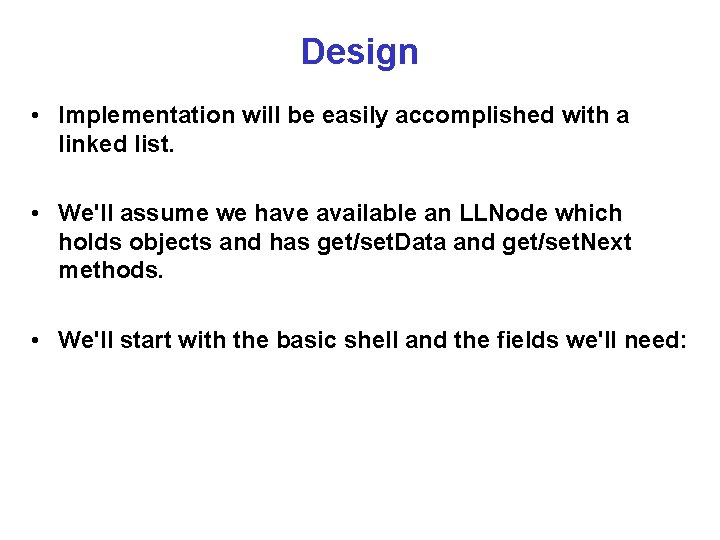Design • Implementation will be easily accomplished with a linked list. • We'll assume we have available an LLNode which holds objects and has get/set. Data and get/set. Next methods. • We'll start with the basic shell and the fields we'll need:LLNode class LLNode { private Object data; private LLNode next; public LLNode(Object o) { set. Data(o); set. Next(null); } public void set. Data(Object o) { data = o; } public Object get. Data() { return data; }LLNode // class LLNode (continued) public void set. Next(LLNode n) { next = n; } public LLNode get. Next() { return next; } } // LLNodeThings to notice • As you get used to any language you will learn a basic shell or template from which to start programs. • Creating and using such a shell will sometimes aid in getting started • It’s also a good place to include things that you always forget (e. g. closing braces)Basics public class Vector { private LLNode head; private int count; /* ---------------------- */ /** Basic constructor. <BR><B>Precondition: </B> None. <BR><B>Postcondition: </B> Vector instantiated with size set to zero. */ public Vector() { set. Head(null); count = 0; }Basics /** Returns head reference. <BR><B>Precondition: </B> Instantiation. <BR><B>Postcondition: </B> No change to Vector @return Head reference */ private LLNode get. Head() { return head; }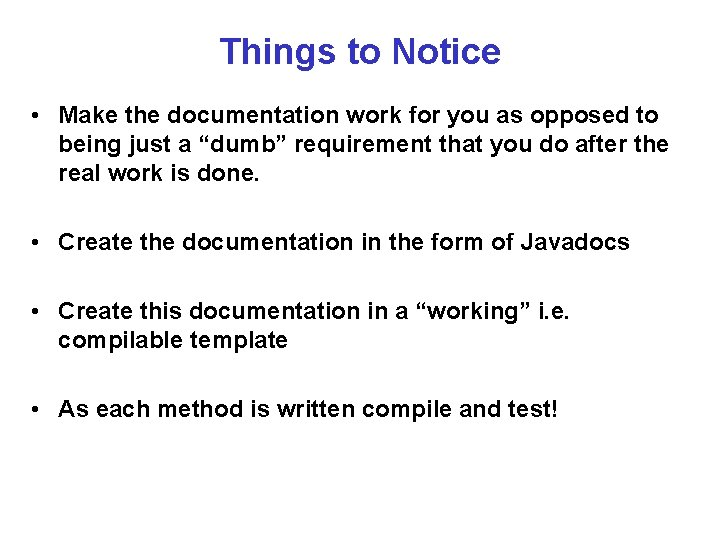Things to Notice • Make the documentation work for you as opposed to being just a “dumb” requirement that you do after the real work is done. • Create the documentation in the form of Javadocs • Create this documentation in a “working” i. e. compilable template • As each method is written compile and test!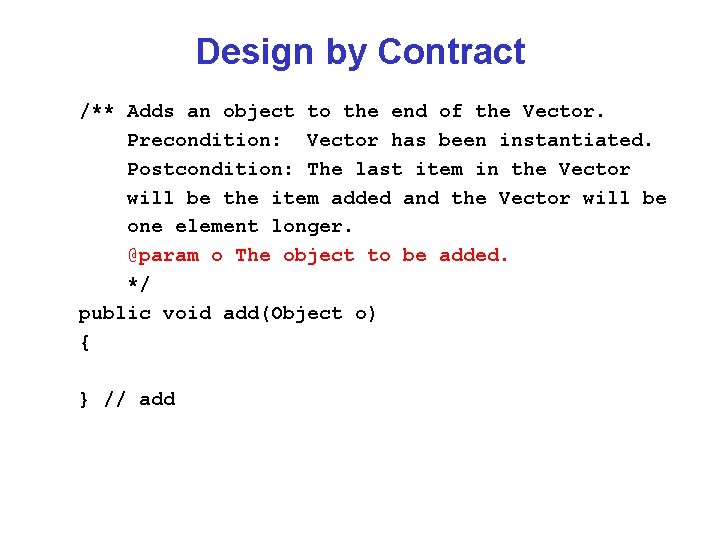Design by Contract /** Adds an object to the end of the Vector. Precondition: Vector has been instantiated. Postcondition: The last item in the Vector will be the item added and the Vector will be one element longer. @param o The object to be added. */ public void add(Object o) { } // add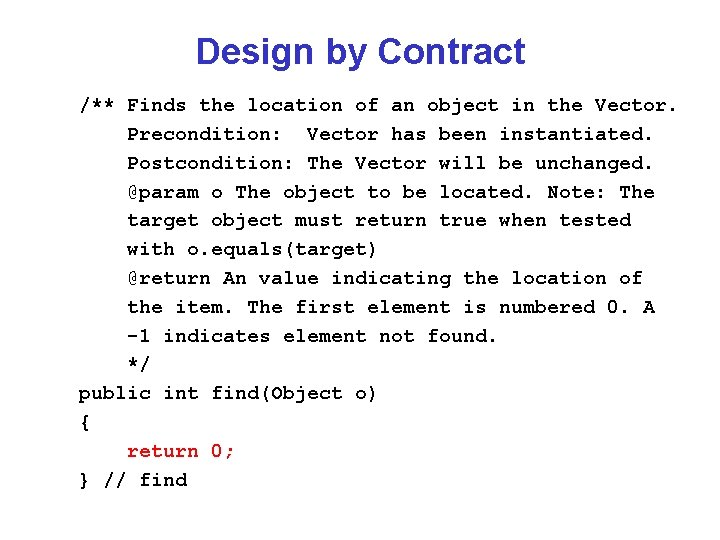Design by Contract /** Finds the location of an object in the Vector. Precondition: Vector has been instantiated. Postcondition: The Vector will be unchanged. @param o The object to be located. Note: The target object must return true when tested with o. equals(target) @return An value indicating the location of the item. The first element is numbered 0. A -1 indicates element not found. */ public int find(Object o) { return 0; } // findDesign by Contract /** Gets the n-th element of the Vector and returns it. Precondition: Vector has been instantiated and should have at least n+1 elements. Postcondition: The Vector will be unchanged. @param n The number of the object to be located. Note: The first object in the Vector is numbered 0. @return The desired object. If the Vector is not at least n+1 elements long null will be returned. */ public Object get(int i) { return null; } // get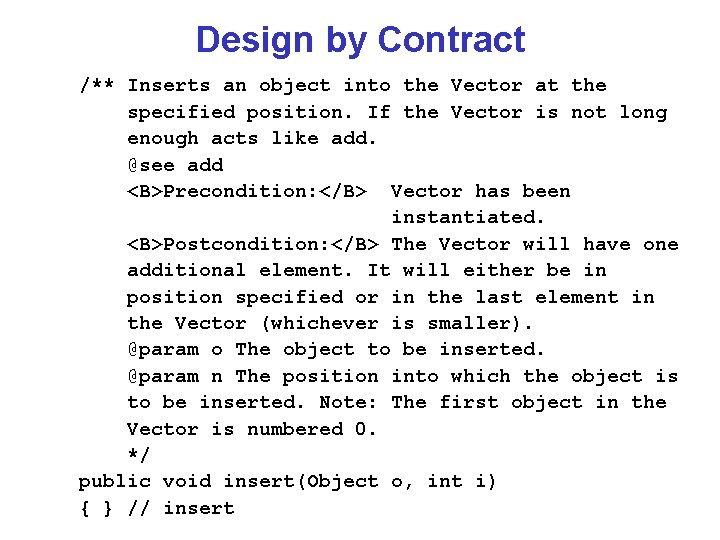Design by Contract /** Inserts an object into the Vector at the specified position. If the Vector is not long enough acts like add. @see add <B>Precondition: </B> Vector has been instantiated. <B>Postcondition: </B> The Vector will have one additional element. It will either be in position specified or in the last element in the Vector (whichever is smaller). @param o The object to be inserted. @param n The position into which the object is to be inserted. Note: The first object in the Vector is numbered 0. */ public void insert(Object o, int i) { } // insert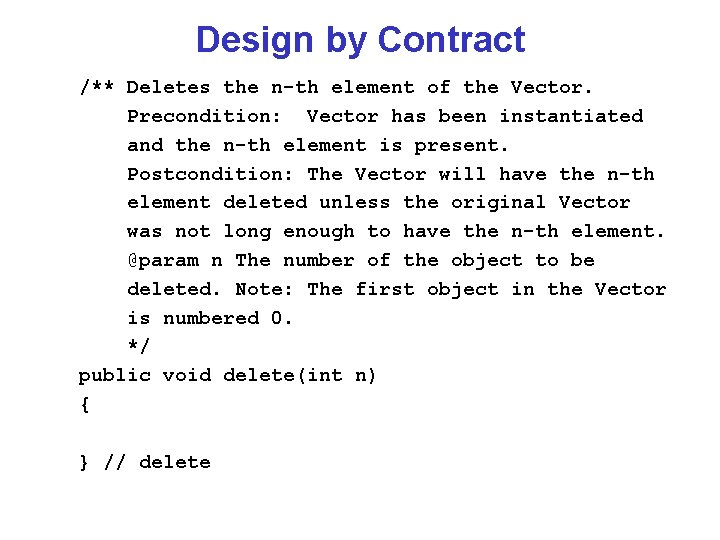Design by Contract /** Deletes the n-th element of the Vector. Precondition: Vector has been instantiated and the n-th element is present. Postcondition: The Vector will have the n-th element deleted unless the original Vector was not long enough to have the n-th element. @param n The number of the object to be deleted. Note: The first object in the Vector is numbered 0. */ public void delete(int n) { } // deleteDesign by Contract /** Returns the size of the Vector. Precondition: Vector has been instantiated. Postcondition: Vector will be unchanged. @return Size of the Vector. */ public int size() { return 0; } // delete } // Vector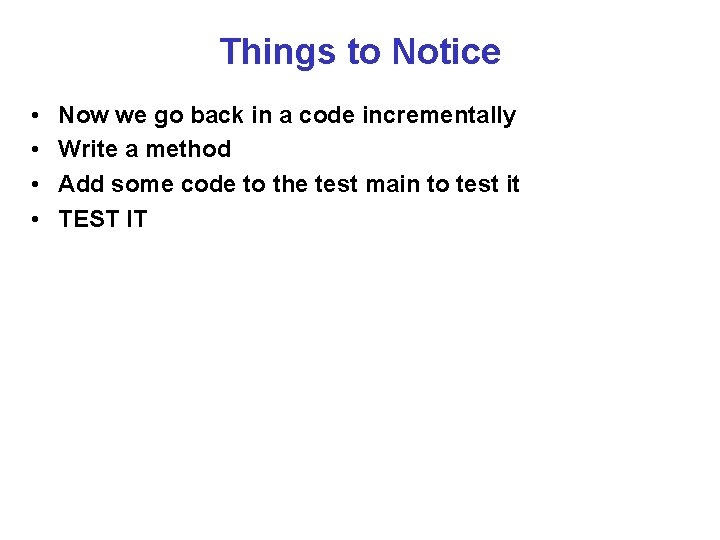Things to Notice • • Now we go back in a code incrementally Write a method Add some code to the test main to test it TEST IT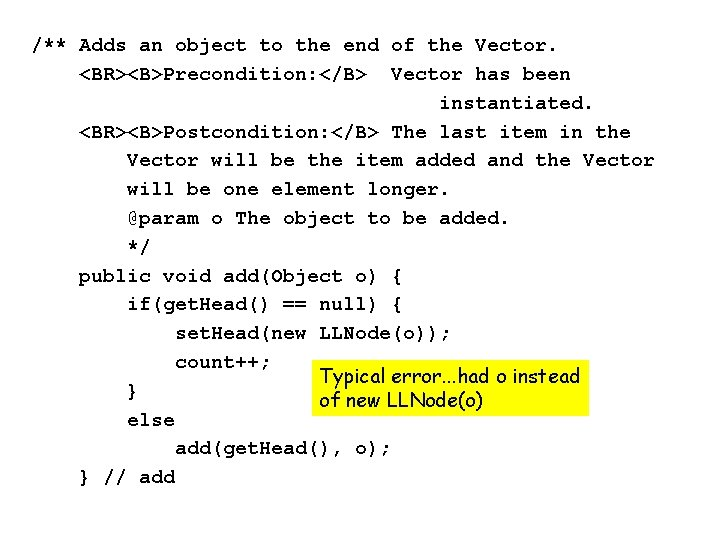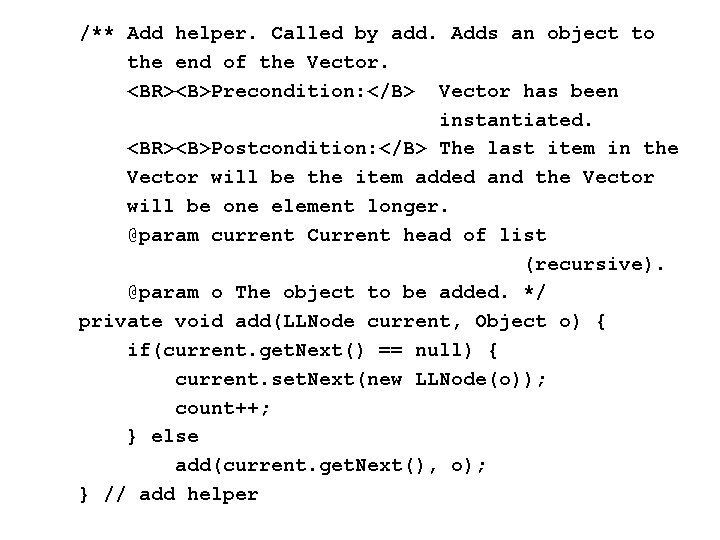/** Add helper. Called by add. Adds an object to the end of the Vector. <BR><B>Precondition: </B> Vector has been instantiated. <BR><B>Postcondition: </B> The last item in the Vector will be the item added and the Vector will be one element longer. @param current Current head of list (recursive). @param o The object to be added. */ private void add(LLNode current, Object o) { if(current. get. Next() == null) { current. set. Next(new LLNode(o)); count++; } else add(current. get. Next(), o); } // add helper/** Finds the location of an object in the Vector. <BR><B>Precondition: </B> Vector has been instantiated. <BR><B>Postcondition: </B> The Vector will be unchanged. @param o The object to be located. Note: The target object must return true when tested with o. equals(target) @return An value indicating the location of the item. The first element is numbered 0. A -1 indicates element not found. */ public int find(Object o) { if(get. Head() == null) return -1; else return find(get. Head(), o, 0); } // find/* -----------------------*/ /* find helper */ /* -----------------------*/ private int find(LLNode current, Object o, int k) { if(current. get. Data(). equals(o)) return k; else if(current. get. Next() == null) return -1; else return find(current. get. Next(), o, k+1); } // find helper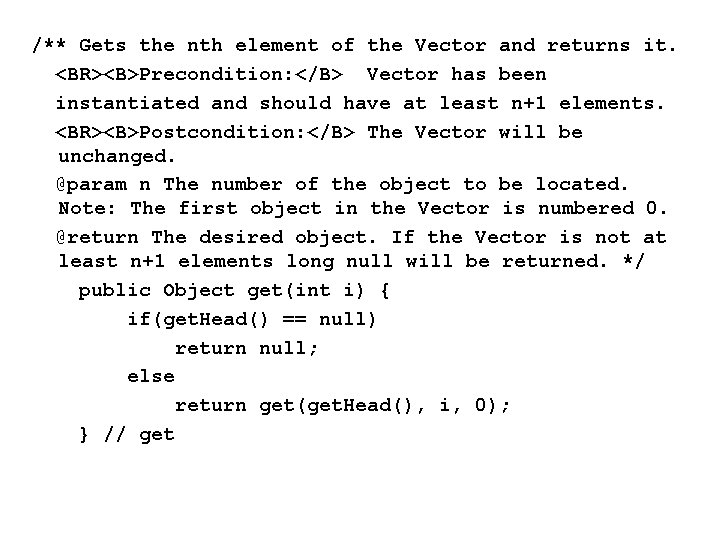/** Gets the nth element of the Vector and returns it. <BR><B>Precondition: </B> Vector has been instantiated and should have at least n+1 elements. <BR><B>Postcondition: </B> The Vector will be unchanged. @param n The number of the object to be located. Note: The first object in the Vector is numbered 0. @return The desired object. If the Vector is not at least n+1 elements long null will be returned. */ public Object get(int i) { if(get. Head() == null) return null; else return get(get. Head(), i, 0); } // get/* -----------------------*/ /* get helper /* -----------------------*/ private Object get(LLNode current, int i, int k) { if(i == k) return current. get. Data(); else if(current. get. Next() == null) return null; else return get(current. get. Next(), i, k+1); } // get helper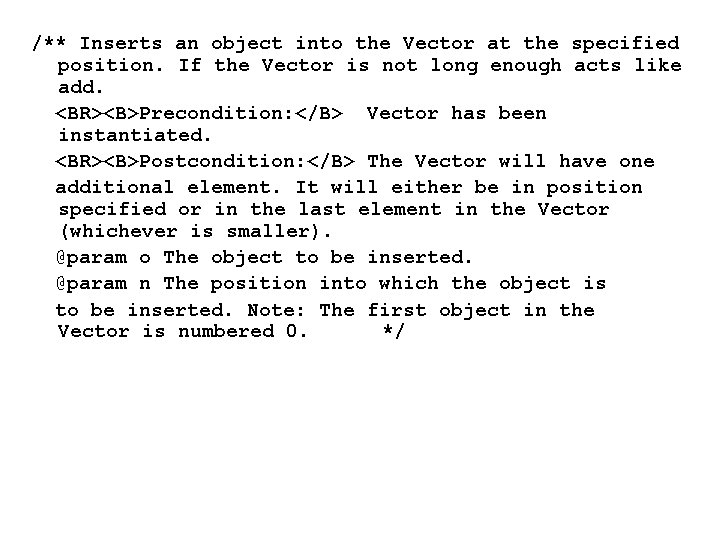/** Inserts an object into the Vector at the specified position. If the Vector is not long enough acts like add. <BR><B>Precondition: </B> Vector has been instantiated. <BR><B>Postcondition: </B> The Vector will have one additional element. It will either be in position specified or in the last element in the Vector (whichever is smaller). @param o The object to be inserted. @param n The position into which the object is to be inserted. Note: The first object in the Vector is numbered 0. */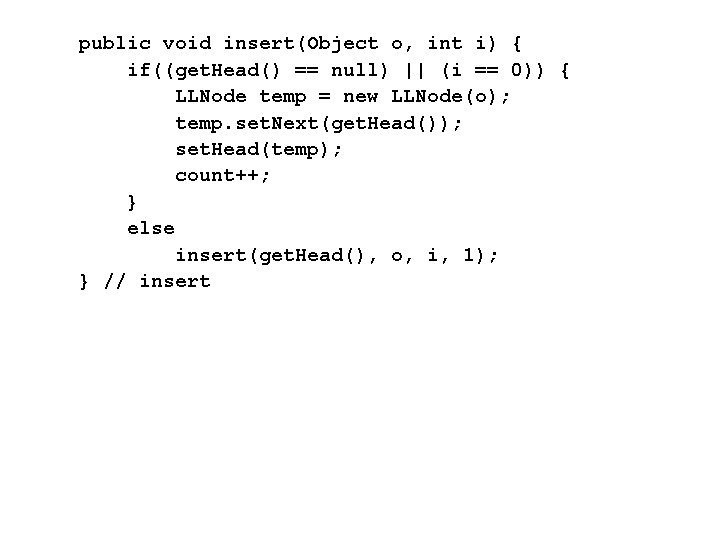public void insert(Object o, int i) { if((get. Head() == null) || (i == 0)) { LLNode temp = new LLNode(o); temp. set. Next(get. Head()); set. Head(temp); count++; } else insert(get. Head(), o, i, 1); } // insert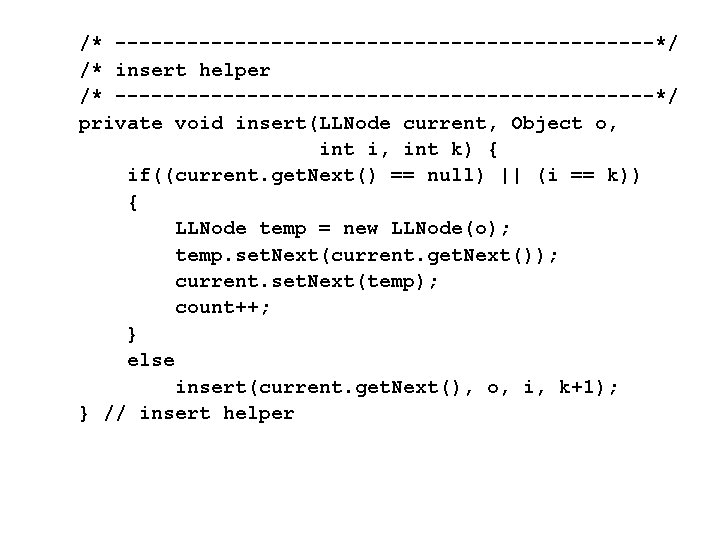/* -----------------------*/ /* insert helper /* -----------------------*/ private void insert(LLNode current, Object o, int i, int k) { if((current. get. Next() == null) || (i == k)) { LLNode temp = new LLNode(o); temp. set. Next(current. get. Next()); current. set. Next(temp); count++; } else insert(current. get. Next(), o, i, k+1); } // insert helper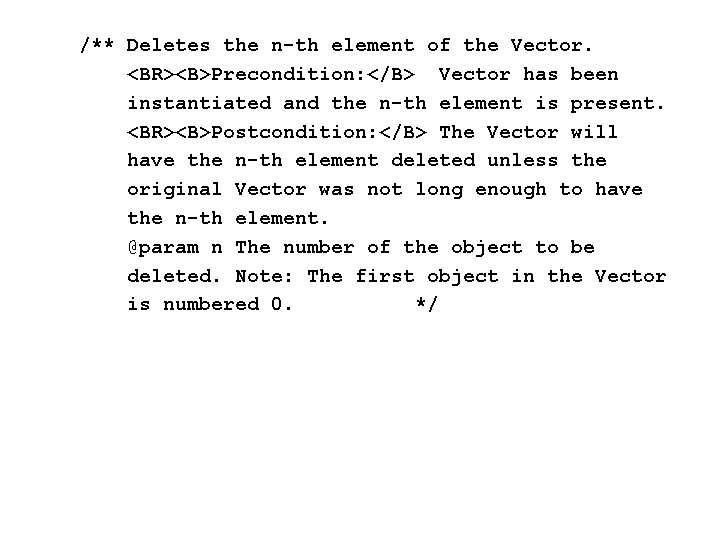/** Deletes the n-th element of the Vector. <BR><B>Precondition: </B> Vector has been instantiated and the n-th element is present. <BR><B>Postcondition: </B> The Vector will have the n-th element deleted unless the original Vector was not long enough to have the n-th element. @param n The number of the object to be deleted. Note: The first object in the Vector is numbered 0. */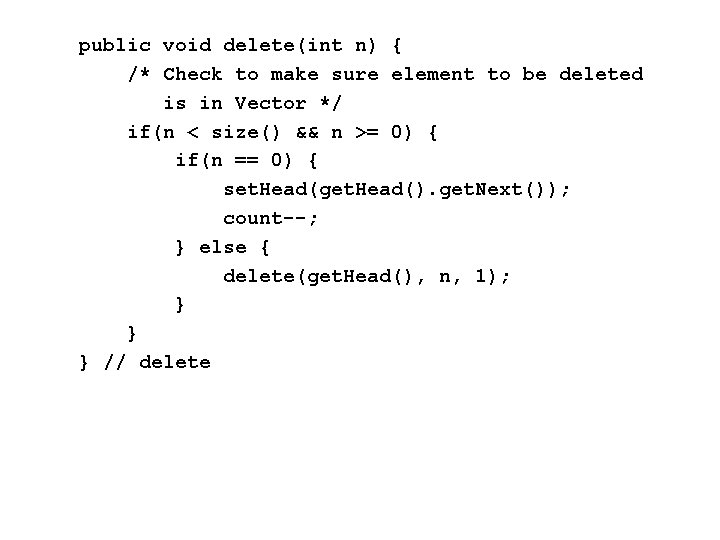public void delete(int n) { /* Check to make sure element to be deleted is in Vector */ if(n < size() && n >= 0) { if(n == 0) { set. Head(get. Head(). get. Next()); count--; } else { delete(get. Head(), n, 1); } } } // delete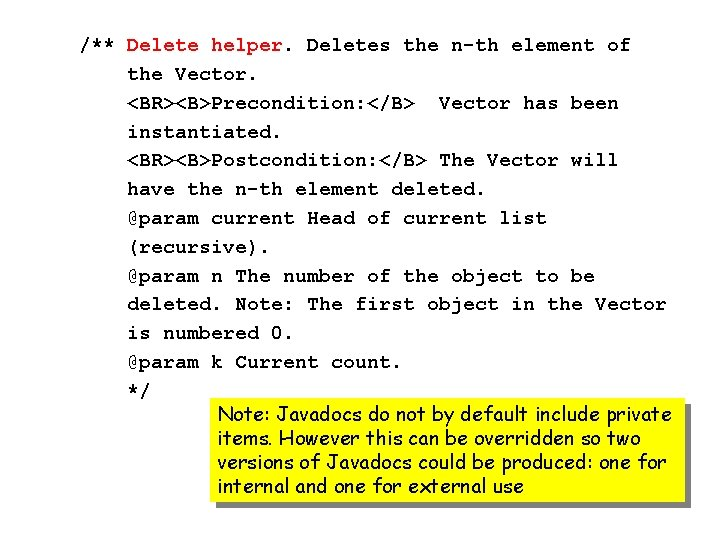/** Delete helper. Deletes the n-th element of the Vector. <BR><B>Precondition: </B> Vector has been instantiated. <BR><B>Postcondition: </B> The Vector will have the n-th element deleted. @param current Head of current list (recursive). @param n The number of the object to be deleted. Note: The first object in the Vector is numbered 0. @param k Current count. */ Note: Javadocs do not by default include private items. However this can be overridden so two versions of Javadocs could be produced: one for internal and one for external use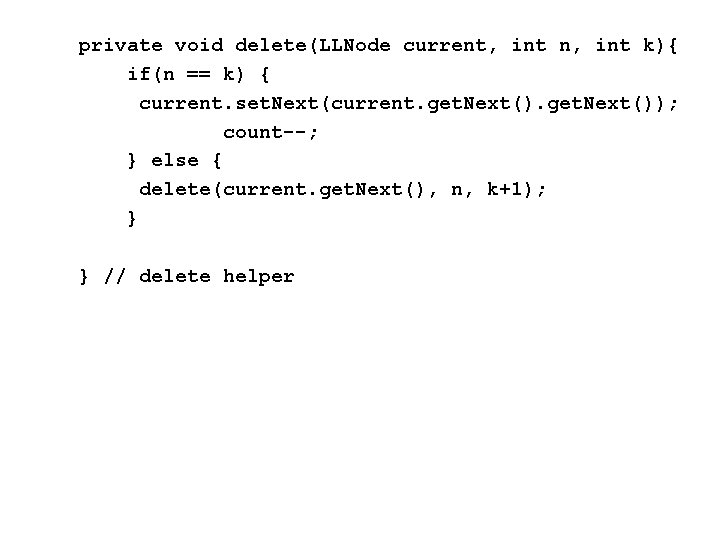private void delete(LLNode current, int n, int k){ if(n == k) { current. set. Next(current. get. Next()); count--; } else { delete(current. get. Next(), n, k+1); } } // delete helper/** Returns the size of the Vector. <BR><B>Precondition: </B> Vector has been instantiated. <BR><B>Postcondition: </B> Vector will be unchanged. @return Size of the Vector. */ public int size() { return count; } // size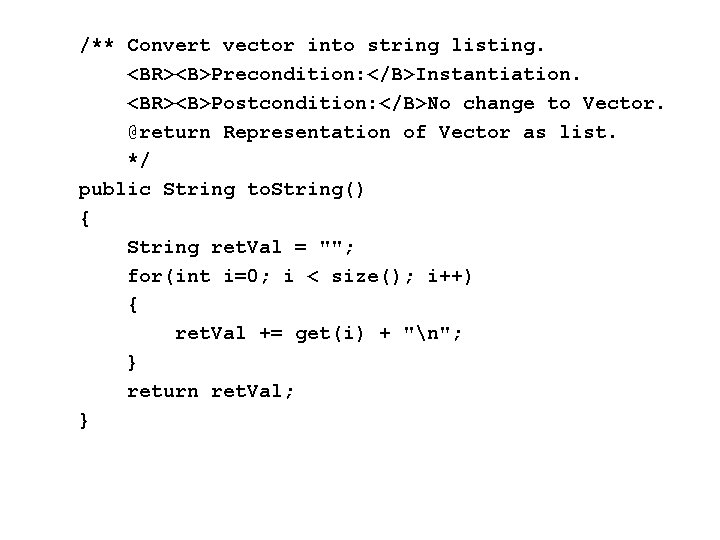/** Convert vector into string listing. <BR><B>Precondition: </B>Instantiation. <BR><B>Postcondition: </B>No change to Vector. @return Representation of Vector as list. */ public String to. String() { String ret. Val = ""; for(int i=0; i < size(); i++) { ret. Val += get(i) + "n"; } return ret. Val; }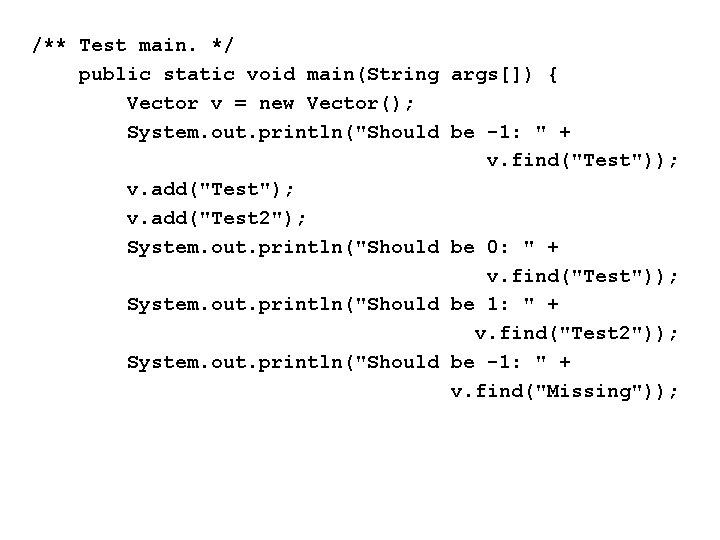/** Test main. */ public static void main(String args[]) { Vector v = new Vector(); System. out. println("Should be -1: " + v. find("Test")); v. add("Test"); v. add("Test 2"); System. out. println("Should be 0: " + v. find("Test")); System. out. println("Should be 1: " + v. find("Test 2")); System. out. println("Should be -1: " + v. find("Missing"));/** Test main. */ /* Test get */ System. out. println("Should be Test: " + v. get(0)); System. out. println("Should be Test 2: " + v. get(1)); if(v. get(2) == null) System. out. println("Passed get 2 test"); else System. out. println("Failed get 2 test");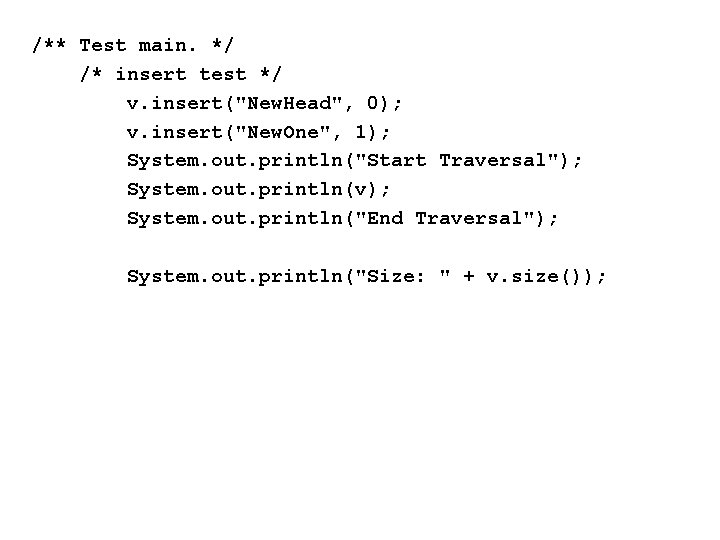/** Test main. */ /* insert test */ v. insert("New. Head", 0); v. insert("New. One", 1); System. out. println("Start Traversal"); System. out. println(v); System. out. println("End Traversal"); System. out. println("Size: " + v. size());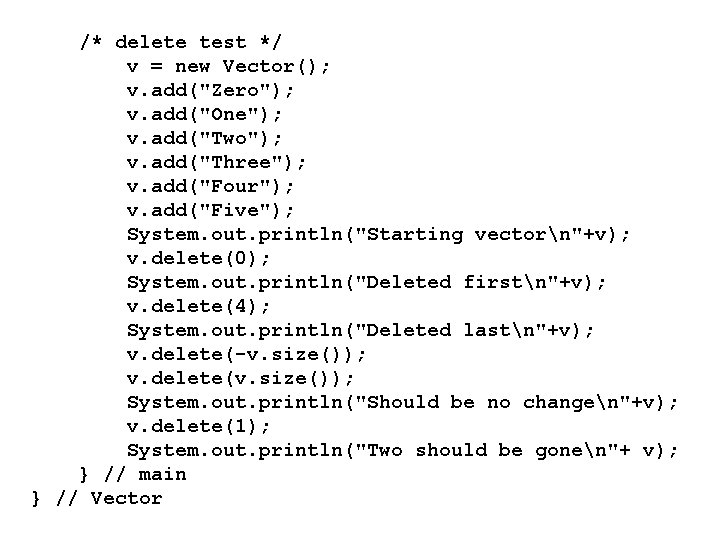/* delete test */ v = new Vector(); v. add("Zero"); v. add("One"); v. add("Two"); v. add("Three"); v. add("Four"); v. add("Five"); System. out. println("Starting vectorn"+v); v. delete(0); System. out. println("Deleted firstn"+v); v. delete(4); System. out. println("Deleted lastn"+v); v. delete(-v. size()); v. delete(v. size()); System. out. println("Should be no changen"+v); v. delete(1); System. out. println("Two should be gonen"+ v); } // main } // Vector